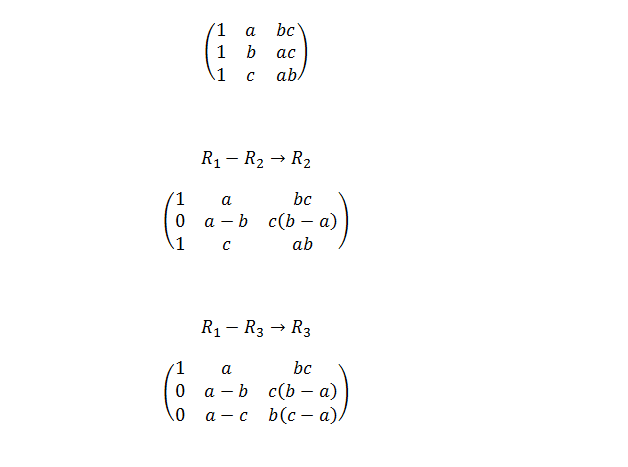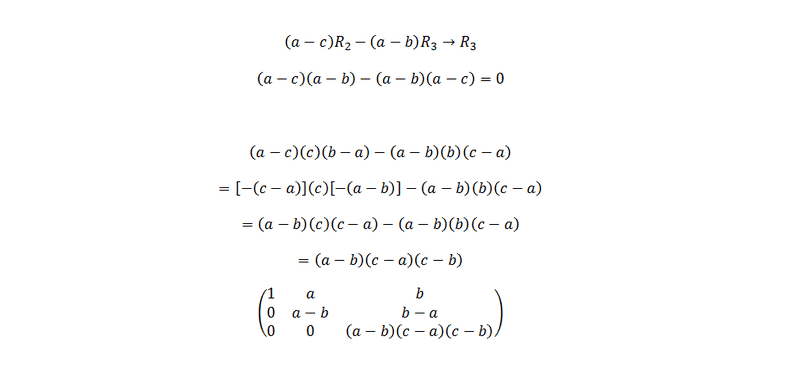# Determinant of a 3x3 matrix via row reduction

## Homework Statement

Show that the determinant ofis (a-b)(b-c)(c-a)

## Homework Equations

Row reduction, determinants

## The Attempt at a SolutionApparently I got a (a-b)^2 instead of (a-b) when I multiplied them up. It would be grateful if someone can point me out where the mistakes are.member 587159
The problem is this. You seem to believe that when you perform a row operation: ##(a-c)R_2 - (a-b)R_3 \rightarrow R3##, the determinant remains unchanged.

To show you an easy example that this is not true:

## A = \begin{pmatrix}
1 & 2 \\
2 & 3
\end{pmatrix}##

It's obvious that this matrix has a determinant equal to ##-1##

Now perform: ##2R_1 + 3R_2 \rightarrow R_2##

Then, we obtain a new matrix A':

## A' = \begin{pmatrix}
1 & 2 \\
8 & 13
\end{pmatrix}##

And this matrix has a determinant equal to ##-3##

Last edited by a moderator:
So any ideas to work it out then?

member 587159
So any ideas to work it out then?

You were on the right track. I will give you this hint:

When you perform a row (kolom) operation:

##R_a + k*R_b \rightarrow R_a##, the determinant remains unchanged.
##l*R_a + R_b \rightarrow R _a##, the determinant is multiplied by ##l##.
##l*R_a + k*R_b \rightarrow R_a##, the determinant is multiplied by ##l##.

Where ##R_a## and ##R_b## are the a'th and the b'th row and ##k,l \in \mathbb{R}##

Now, keep in mind that you had something of the form ##l*R_a + k*R_b \rightarrow R_a##, so your determinant is multiplied with ##-(a-b)##. To make sure that the equality will still hold, multiply the determinant with the factor ##\frac{-1}{a-b}##.

Last edited by a moderator:
PeroK
Homework Helper
Gold Member
2020 Award
So any ideas to work it out then?

Why bother with row operations? Why not evaluate the determinant as it is and simplify?

member 587159
Why bother with row operations? Why not evaluate the determinant as it is and simplify?

I supppose that's the exercise since it's in the title...

PeroK